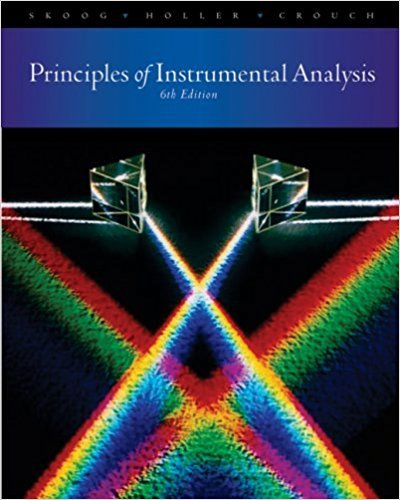×
×

# Calculate the theoretical reciprocal linear dispersion of an echelle grating witha focalISBN: 9780495012016 317

## Solution for problem 10-4 Chapter 10

Principles of Instrumental Analysis | 6th Edition

• Textbook Solutions
• 2901 Step-by-step solutions solved by professors and subject experts
• Get 24/7 help from StudySoup virtual teaching assistantsPrinciples of Instrumental Analysis | 6th Edition

4 5 1 288 Reviews
17
1
Problem 10-4

Calculate the theoretical reciprocal linear dispersion of an echelle grating witha focal length of O.S5m, a groove density of 120 grooves/mm, and a diffractionangle of 6326' when the diffraction order is (a) 30 and (b) 90.

Step-by-Step Solution:
Step 1 of 3

Chap 13: The Properties of Mixture; Solution and Colloids Mixture ­2+ substances mixed together physically ­composition is variable ­retains some properties of its components Colloid (Heterogeneous Mixture) ­has 1+ visible boundaries between its components ­composition is NOT uniform; varies from region to region Solution (Homogeneous Mixture) ­no visible boundaries b/c particles are individual...

Step 2 of 3

Step 3 of 3

##### ISBN: 9780495012016

Principles of Instrumental Analysis was written by and is associated to the ISBN: 9780495012016. This textbook survival guide was created for the textbook: Principles of Instrumental Analysis , edition: 6. The answer to “Calculate the theoretical reciprocal linear dispersion of an echelle grating witha focal length of O.S5m, a groove density of 120 grooves/mm, and a diffractionangle of 6326' when the diffraction order is (a) 30 and (b) 90.” is broken down into a number of easy to follow steps, and 36 words. Since the solution to 10-4 from 10 chapter was answered, more than 232 students have viewed the full step-by-step answer. This full solution covers the following key subjects: . This expansive textbook survival guide covers 34 chapters, and 619 solutions. The full step-by-step solution to problem: 10-4 from chapter: 10 was answered by , our top Chemistry solution expert on 03/02/18, 06:21PM.

Unlock Textbook Solution